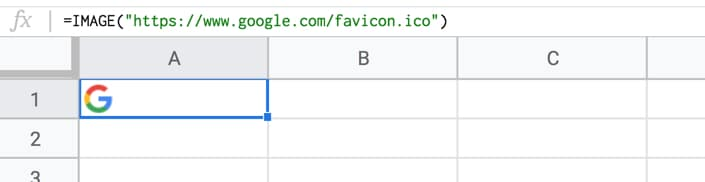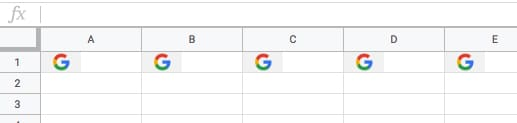# Formula Challenge #1: Repeating Images with Array Formulas

## Introduction

I firmly believe that one of the most effective and rewarding ways to learn a skill is through practical application.

Solving problems you don’t know the answer to is arguably the best way to do this.

And that’s the idea behind these Formula Challenges.

I’ll post a challenge in my Monday newsletter — a question to be solved with formulas in Google Sheets — and a week later share solutions, both my own and those submitted by readers.

I’ll archive the challenges and solutions on my website here.

This first Formula Challenge originally appeared in my Google Sheets Tips newsletter, on 25 February 2019.

Sign up here so you don’t miss out on future Formula Challenges:

## The Challenge

`=IMAGE("https://www.google.com/favicon.ico")`Your challenge is to modify the formula in cell A1 only, to repeat the image across multiple columns (say 5 as in this example), so it looks like this:Rules

You’re only allowed to use a single formula in cell A1.

The problem is that the IMAGE function can’t be nested inside a REPT function, so you have to get a bit more creative.

## The Solution

### Solution One: using ROW or COLUMN counts

`=ArrayFormula(IF(COLUMN(A:E),IMAGE("https://www.google.com/favicon.ico")))`

#### How does this formula work?

The combination of ArrayFormula with COLUMN(A:E) will output an array of numbers 1 to 5: `{1,2,3,4,5}`

The IF statement treats the numbers as TRUE values, so prints out the image 5 times. For brevity, we can omit the FALSE value of the IF statement, since we don’t call it.

### Solution Two: using REPT inside the IMAGE formula!

`=ArrayFormula(IMAGE(SPLIT(REPT("https://www.google.com/favicon.ico"&"|",5),"|")))`

#### How does this formula work?

As mentioned, the REPT function doesn’t work when wrapped around the IMAGE function. However, flip them around, with the REPT inside the IMAGE function, and it does work!

In other words the IMAGE function accepts arrays of URLs as an input.

Start with this formula in cell A1, which creates a single string of joined URLs, with a pipe ( | ) delimiter between them:

`=ArrayFormula(REPT("https://www.google.com/favicon.ico"&"|",5))`

Now, split these into an array of 5 separate URLs:

`=ArrayFormula(SPLIT(REPT("https://www.google.com/favicon.ico"&"|",5),"|"))`

Finally, wrap this with the IMAGE function to get the five images in a row:

`=ArrayFormula(IMAGE(SPLIT(REPT("https://www.google.com/favicon.ico"&"|",5),"|")))`

What I like about this solution is that you could put the number 5 into a different cell and reference it, so that you can easily change how many times the image is repeated.

You could even embed another formula to calculate how many times to repeat the image 😉

See the other Formula Challenges here.

## 4 thoughts on “Formula Challenge #1: Repeating Images with Array Formulas”

1.BERTRAND JAMES says:

FANTASTIC FORMULAS

2.Colin says:

What if there was a section you wanted to repeat based on a value in a cell – is that possible?

Say I wanted to repeat A9-L10 by the amount in cell B6. The values contain text and numbers.

3.Ted Haller says:

Sum (1+g)^n/(1+k)^n
Where n=year

When I put this formula In google sheets it sums all the numerators and all the denominators and then divides the two totals.

I want it to calculate year 1,2,3,4,….. and then sum. Can not figure out how to make this work. Tried extra parentheses etc.
Can anyone help?

1.Wilson says:

=arrayformula (SUM((1+A1:A3)^2/(1+B1:B3)^2))

i think this is what you need